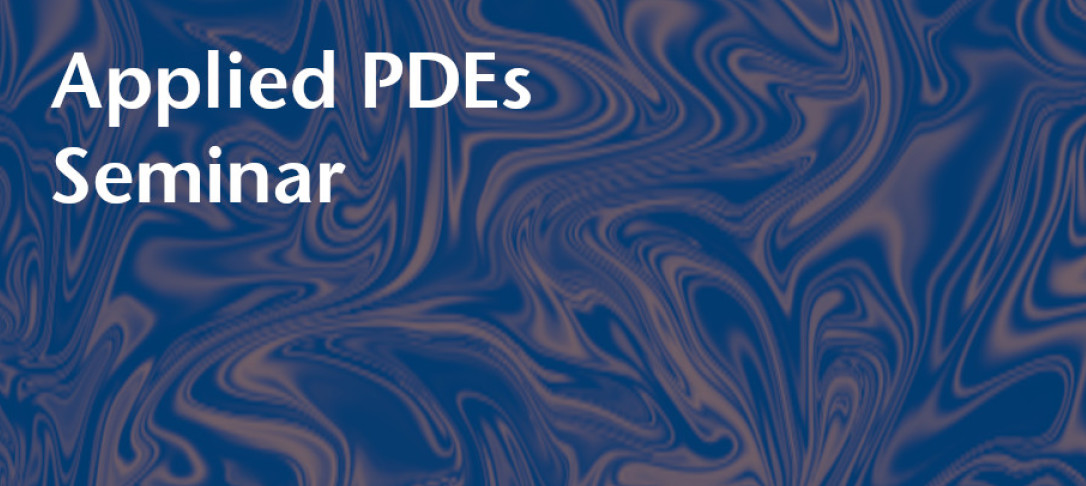We consider the sharp interface limit of a Navier-Stokes/Allen-Cahn system, when a parameter $\varepsilon>0$ that is proportional to the thickness of the diffuse interface tends to zero, in a two dimensional bounded domain. We present a recent result in which we prove convergence for sufficiently small times of the solutions of the Navier-Stokes/Allen-Cahn system to solutions of a sharp interface model, where the interface evolution is given by the mean curvature equation with an additional convection term coupled to a two-phase Navier-Stokes system with an additional contribution to the stress tensor, which describes the capillary stress. To this end we construct a suitable approximation of the solution of the Navier-Stokes/Allen-Cahn system. To this end a new Ansatz based on partial linearization in highest order is used, which simplifies the analysis compared to previous results. Then the difference of approximate and exact solution is estimated with the aid of a suitable spectral estimate of the linearized Allen-Cahn operator. This is a joint work with Mingwen Fei from Anhui Normal University, China.